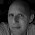## Tuesday, January 4, 2011

### Clojure versus Scala (part 1)

This is just a brain dump, after having read an excellent article on Clojure by Maurits and Sander in the Dutch Java magazine. Admittedly, without having access to the original article, it probably isn't of any use, but I just wanted to jot it down here, for future reference.

## Hello World

Let's start with their simple Hello world example. This is the Clojure version:

```(def hello (fn [target] (println (str "Hello " target))))
(hello "Clojure")
```

or the short version:

```(defn hello [target] (println (str "Hello " target)))
(hello "Clojure")
```

... and this is the Scala version - even shorter:

```def hello(target: Any) = println("Hello " + target)
hello("Scala")
```

I'm happy to say that the Scala version is shorter in number of characters, even though the type of parameter 'target' had to be specified explicitly.

## Doc String

Next they explain the purpose of the doc string. Now this is something that I truly miss in Scala. It would be totally awesome to have the ability to pull up documentation on a function from the REPL, but it doesn't exist. Scala does have scaladoc, but that's all thrown away at compilation time. It should be possible to store some of this in AttributeInfo, but it doesn't.

This is clearly an area in which Clojure's ancestry of LISP and Scala's ancestry of Java shows.

## First class functions

Before going any further, we first need to have square function, as defined in the article like this:

```(defn square [x] (* x x))
```

Now, I would love to say that defining square in Scala is just as easy. If you would only define square for integers, it would be:

```def square(x: Int) = x * x
```

... but we obviously want it to work for doubles as well, as I suspect the Clojure version does.

Now, this is the Scala version that supports any numeric type:

```def square[T](x: T)(implicit numeric: Numeric[T]): T =
numeric.times(x, x)
```

in Scala 2.8.1 there is a shorthand notation for the same thing:

```def square[T: Numeric](x: T) =
implicitly[Numeric[T]].times(n, n)```

Not quite as intuitive as you would have expected, but it works quite well:

```scala> square(4.0)
res1: Double = 16.0

scala> square(5)
res2: Int = 25
```

Once the square function has been defined, the article explains that functions like square could be passed to a function called twice, with twice being defined like this:

```(defn twice [f a] (f (f a)))
(twice square 2)
```

This is the Scala version:

```def twice[T](a: T)(f: (T) => T) = f(f(a))
twice(4)(square)
```

It may look a little awkward at first, but this is the only way you get it to work without having to pass in additional type information. (Check this for more information.)

Here's an alternative that does not work:

```scala> def twice[T](a: T, f: (T) => T) = f(f(a))
twice: [T](a: T,f: (T) => T)T

scala> twice(4, square)
:8: error: could not find implicit value for evidence parameter of type Numeric[T]
twice(4, square)
^
```

If you insist on defining twice like this - perhaps because of its resemblance to the Clojure version, then the only option you have is call twice like this:

```scala> twice(square[Int], 2)
res70: Int = 16
```

## Data structures

Onward to lists. Clojure example to produce a list:

```(list 1 2 3)
```

... and then a couple of alternatives for doing a Scala List:

```List(1, 2, 3)
1 :: 2 :: 3 :: nil
```

Adding an item to a list in Clojure:

```(conj (list 1 2 3) 4)
```

... and the same in Scala:

```4 :: List(1, 2, 3)
```

In Scala, Lists are typed. You can only add anything to a List if it's a List[Any]. So, this is fine:

```scala> 1 :: "a" :: 2 :: "b" :: Nil
res74: List[Any] = List(1, a, 2, b)

scala> List(1, "a", 2, "b")
res75: List[Any] = List(1, a, 2, b)
```

While Clojure does not allow you to directly access one of the elements unless you use a vector, Scala does allow you to get the nnth element of a list:

```scala> val list = List(1, "a", 2, "b")
list: List[Any] = List(1, a, 2, b)

scala> list(0)
res80: Any = 1

scala> list(2)
res81: Any = 2

scala> list(1)
res82: Any = a```

Now, even though Scala does allow you to do it - that doesn't mean you should feel encouraged to code like that; it also defines a Vector, which is - just like the one in Clojure - way more optimized for random access.

## Maps

This is the Clojure version of defining a map:

```(def mymap '{:aap "monkey" :ezel "donkey" :walvis "whale" :onbekend "platypus"})

(:ezel mymap)
```

... and the Scala version:

```val mymap = Map("aap"->"monkey",
"ezel"->"donkey",
"walvis"->"whale",
"onbekend"->"platypus")
mymap("ezel")
```

Updating the map is a little different than what you are used to, if you come from the Java space. In fact, in that sense, it's not unlike Clojure. In Clojure, you update a map like this:

```(assoc mymap :onbekend "unknown")
```

In Scala it's done slight differently, but the net effect is the same: a new map, containing all of the previously defined entries plus a new one.

```scala> mymap + ("onbekend" -> "unknown")
res90: scala.collection.immutable.Map[java.lang.String,java.lang.String] = Map((aap,monkey), (ezel,donkey), (walvis,whale), (onbekend,unknown))
```

#### 2 comments:

1."While Clojure does not allow you to directly access one of the elements"

You can use nth on any sequence:

(nth (range 100) 42)

gives 42

2.Ah, the article gives the impression that it's not supported.